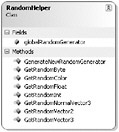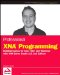# RandomHelper Class

Take a look at one of the helper classes. RandomHelper will not be used often in a single project, but almost any game uses some random number generation to make the game content appear less periodic and to add more variation to the game.

In the Breakout game you will write in a little bit, the blocks are generated randomly. For level 1 you use a probability of 10%, level 2 uses a probability of 20%, and so on. This way the level gets more filled and the game gets harder. You could just use the Random class and call the Next method to get a new random number, but in case you want to generate a random normalized vector you would have to write the following lines of code:

`  Random randomGenerator = new Random((int)DateTime.Now.Ticks); Vector3 randomNormalVector = new Vector3(   (float)randomGenerator.NextDouble() * 2.0f - 1.0f,   (float)randomGenerator.NextDouble() * 2.0f - 1.0f,   (float)randomGenerator.NextDouble() * 2.0f - 1.0f); randomNormalVector.Normalize(); `

Instead of repeating this code over and over again, a helper class like RandomHelper might be very useful. Figure 3-8 shows the basic layout of the RandomHelper class.Figure 3-8

As you can see the methods are very simple and it would only take a couple of minutes to write the whole class. However, the class is still useful and thanks to the internal globalRandomGenerator instance of the Random class, the RandomHelper class is much quicker generating random values than creating a new Random class every time you need a random number.

### Generate Random Vectors

Here you can see a method from the RandomHelper class:

`  /// <summary> /// Get random Vector2 /// </summary> /// <param name="min">Minimum for each component</param> /// <param name="max">Maximum for each component</param> /// <returns>Vector2</returns> public static Vector2 GetRandomVector2(float min, float max) {   return new Vector2(     GetRandomFloat(min, max),     GetRandomFloat(min, max)); } // GetRandomVector2(min, max) `

It does not make sense to unit test any method in the RandomHelper class because all return values are random and you don’t really have to check if GetRandomVector2 returns a Vector2; it just does that. There is not much that can go wrong.Professional XNA Programming: Building Games for Xbox 360 and Windows with XNA Game Studio 2.0
ISBN: 0470261285
EAN: 2147483647
Year: 2007
Pages: 138

Similar book on Amazon

flylib.com © 2008-2017.
If you may any questions please contact us: flylib@qtcs.net